1.生成“表头”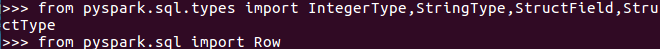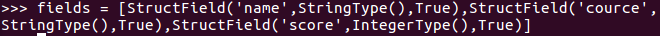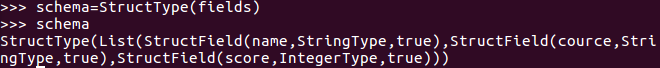2.生成“表中的记录”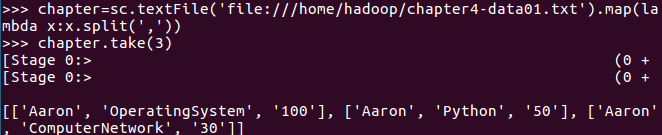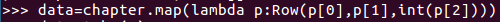3.把“表头”和“表中的记录”拼装在一起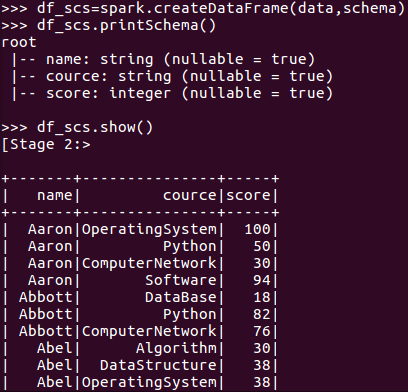• 每个分数+5分。
• df_scs.select('name','cource',df_scs['score']+5).show()
•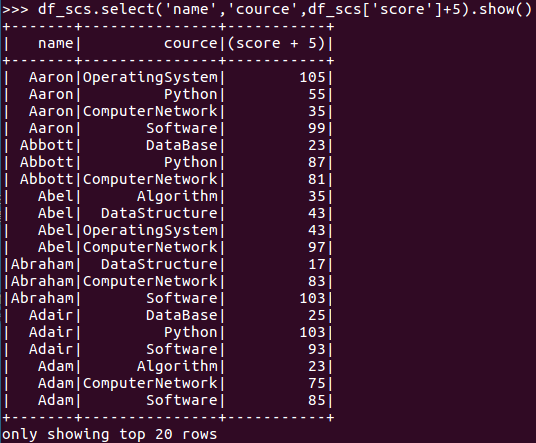• 总共有多少学生？
• df_scs.select('name').distinct().count()
•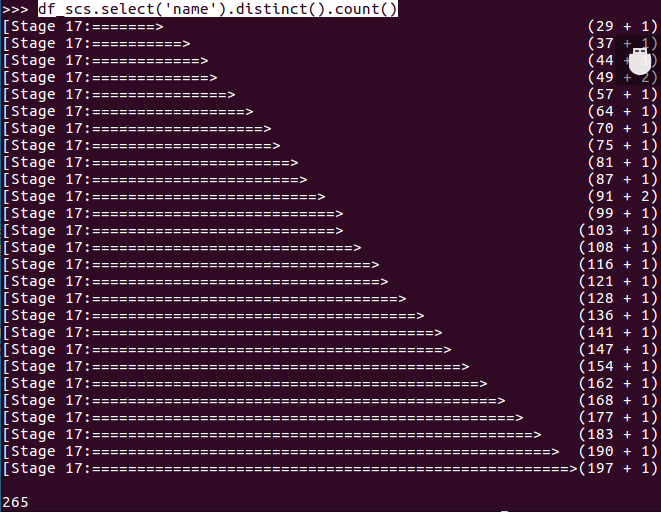• 总共开设了哪些课程？
• df_scs.select('cource').distinct().show()
•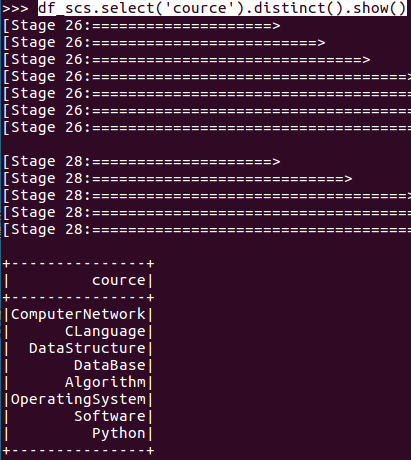• 每个学生选修了多少门课？
• df_scs.groupBy('name').count().show()
•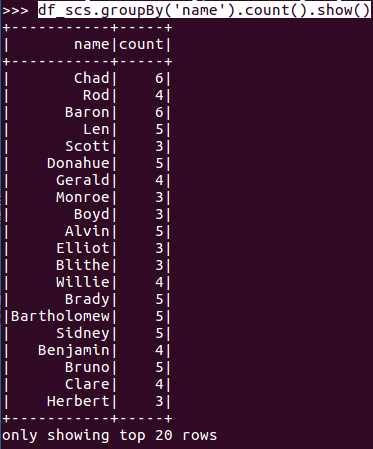• 每门课程有多少个学生选？
• df_scs.groupBy('cource').count().show()
•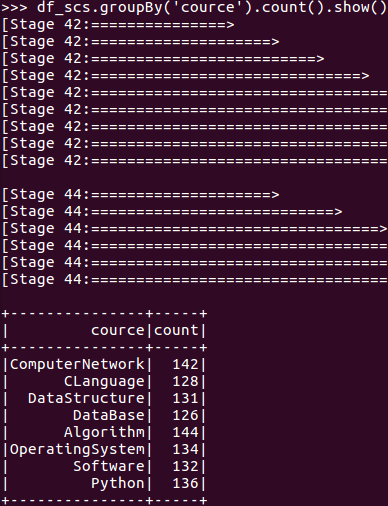• 每门课程大于95分的学生人数？
• df_scs.filter(df_scs['score']>95).groupBy('cource').count().show()
•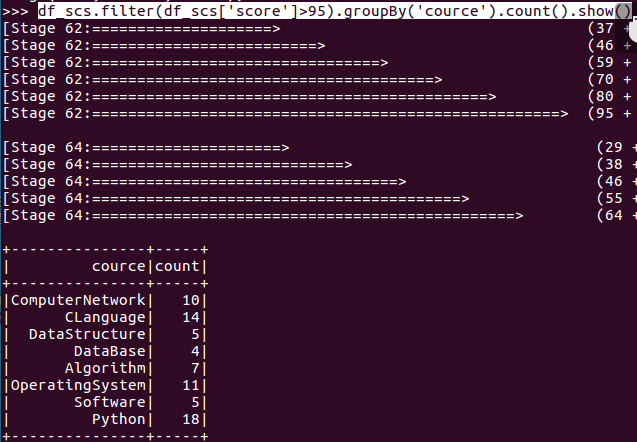• Tom选修了几门课？每门课多少分？
• df_scs.filter(df_scs['name']=='Tom').select('cource','score').show()
•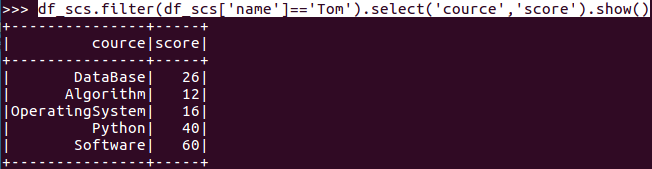• Tom的成绩按分数大小排序。
• df_scs.filter(df_scs['name']=='Tom').sort(df_scs['score'].desc()).show()
•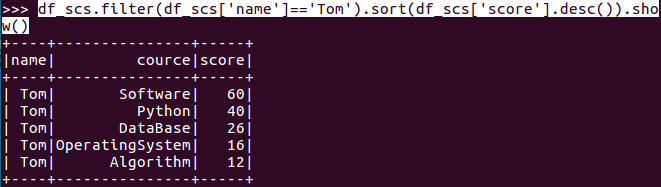• Tom的平均分
• df_scs.filter(df_scs['name']=='Tom').groupBy('cource').avg('score').show()

•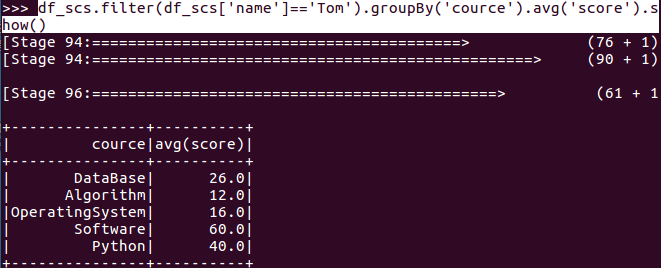• 求每门课的平均分，最高分，最低分。
• df_scs.groupBy("cource").avg('score').show()
• df_scs.groupBy("cource").max('score').show()
• df_scs.groupBy("cource").min('score').show()
•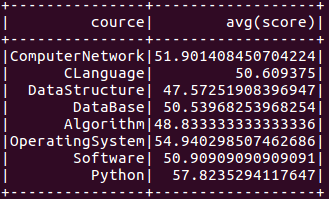•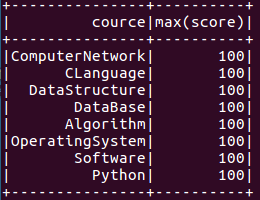•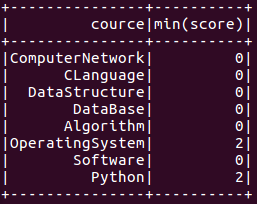• 求每门课的选修人数及平均分，精确到2位小数。
• df_scs.select(countDistinct('name').alias('学生人数'),countDistinct('cource').alias('课程数'),round(mean('score'),2).alias('所有课的平均分').alias('所有课的平均分')).show()
•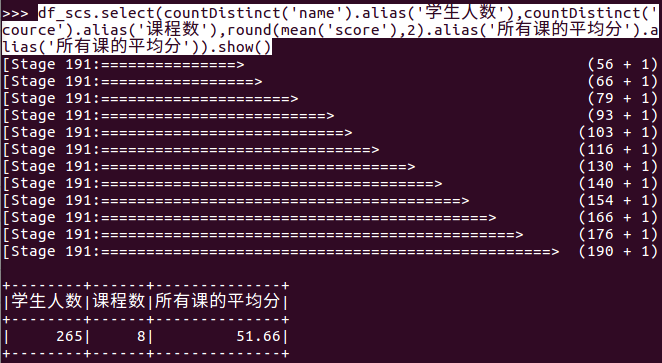• 每门课的不及格人数，通过率
• 结果可视化。
posted on 2021-05-20 19:15  桉叶  阅读(77)  评论(0编辑  收藏  举报